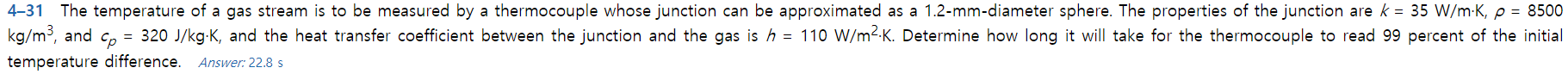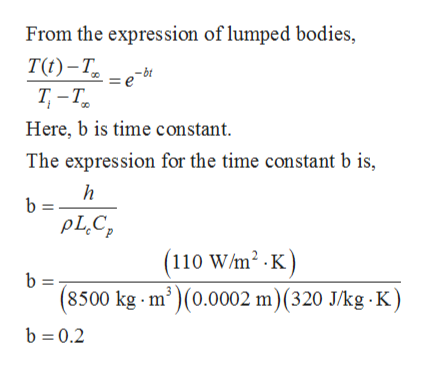# 4-31 The temperature of a gas stream is to be measured by a thermocouple whose junction can be approximated as a 1.2-mm-diameter sphere. The properties of the junction are k 35 W/m K, p = 8500kg/m3, and c, = 320 J/kg-K, and the heat transfer coefficient between the junction and the gas is h = 110 W/m2-K. Determine how long it will take for the thermocouple to read 99 percent of the initialtemperature difference.Answer: 22.8 s

Question
143 viewshelp_outlineImage Transcriptionclose4-31 The temperature of a gas stream is to be measured by a thermocouple whose junction can be approximated as a 1.2-mm-diameter sphere. The properties of the junction are k 35 W/m K, p = 8500 kg/m3, and c, = 320 J/kg-K, and the heat transfer coefficient between the junction and the gas is h = 110 W/m2-K. Determine how long it will take for the thermocouple to read 99 percent of the initial temperature difference. Answer: 22.8 s fullscreen
check_circle

Step 1

The characteristics length of the thermocouple is,

Step 2

The Biot number for the thermocouple sphere is,

Step 3

The Biot number of the sphere is less than <0.1. Thu...help_outlineImage TranscriptioncloseFrom the expression of lumped bodies, TO) -т, Т -Т. Here, b is time constant bt The expression for the time constant b is, b = pLC (110 W/m2 .K) b = (8500 kg m')(0.0002 m) (320 J/kg K) b 0.2 fullscreen

### Want to see the full answer?

See Solution

#### Want to see this answer and more?

Solutions are written by subject experts who are available 24/7. Questions are typically answered within 1 hour.*

See Solution
*Response times may vary by subject and question.
Tagged in

### Heat Transfer